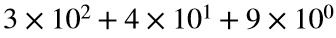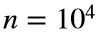# Problem 54775. Flip the parity of digits in a decimal expansion

The number 349 has the decimal expansion. If we change the even digits from positive to negative, then the number becomes 269 (i.e., 300-40+9). A similar operation could be applied to the odd digits.
Write a function that takes a number n and produces two arrays: a vector a with the even digits of 1 to n with flipped parity and a vector b with the odd digits of 1 to n with flipped parity.
At first these sequences did not seem interesting, but plotting them--using plot(a,1:n,b,1:n) with, say—convinced me that they are.

### Solution Stats

100.0% Correct | 0.0% Incorrect
Last Solution submitted on Sep 10, 2022

### Community Treasure Hunt

Find the treasures in MATLAB Central and discover how the community can help you!

Start Hunting!